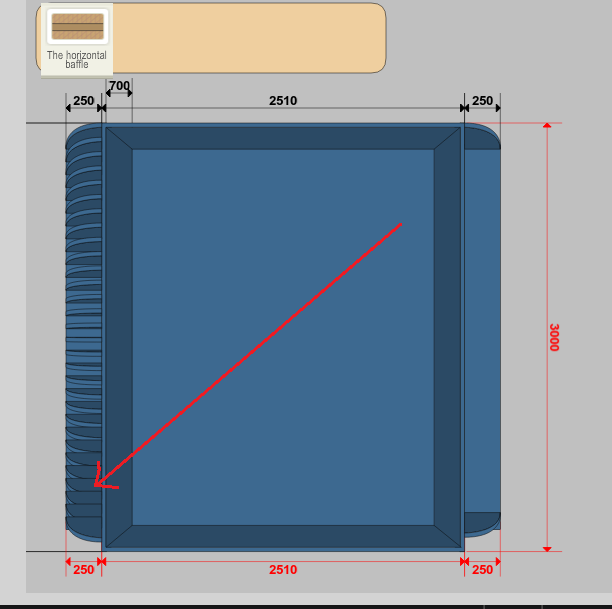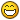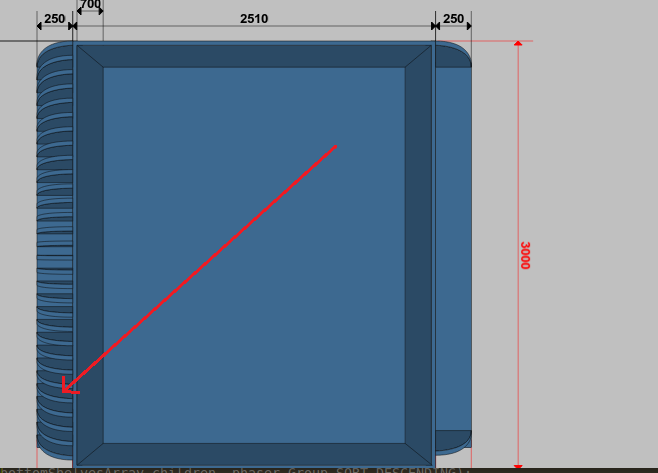# Sort by "y" coordinate, children of Phaser.Graphics object

## Recommended Posts

I just found there is a function called sort(key, order) inside game.world inherited from Phaser.Group.

I have a list of objects of type Phaser.Graphics inside my parent also of type Phaser.Graphics.

The parent object is a wall and the children are shelves. I need to fake a 3D effect that the shelves sit on top of each other as the "y" coordinate increases. I can do this by setting the child index to descending order i.e from larger to smaller, but I need first to sort my shelves by "y" coordinate in ascending order. (The shelves which have the smallest "y" coord. have the highest z-index i.e sit on top of the other).

Could I use this Phaser.Group.sort(key order) function for children of Phaser.Graphics or not?

Or maybe there is a another way to sort graphical objects?##### Share on other sites

Maybe should have treated my parent object as a group instead. Not sureGroups don't have input and i need to drag my parent graphics object.

Never mind, gonna make my own sorting function``````var bubleSort = function(array, sortType) {
var i = 0,
temp = 0,
swapped = false;

do {
swapped = false;
for (i = 0; i < array.length - 1; i += 1) {

if (sortType === Phaser.Group.SORT_ASCENDING) {
if (array[i] > array[i + 1]) {
temp = array[i];
array[i] = array[i + 1];
array[i + 1] = temp;
swapped = true;
}
}

if (sortType === Phaser.Group.SORT_DESCENDING) {
if (array[i] < array[i + 1]) {
temp = array[i];
array[i] = array[i + 1];
array[i + 1] = temp;
swapped = true;
}
}

}
} while (swapped);

return array;
};``````

##### Share on other sites

Looking good``````var graphicsBubleSort = function(prop, grObjectsArray, sortType) {
var i = 0,
temp = null,
swapped = false;

do {
swapped = false;
for (i = 0; i < grObjectsArray.length - 1; i += 1) {

if (sortType === Phaser.Group.SORT_ASCENDING) {
if (grObjectsArray[i][prop] > grObjectsArray[i + 1][prop]) {
temp = grObjectsArray[i];
grObjectsArray[i] = grObjectsArray[i + 1];
grObjectsArray[i + 1] = temp;
swapped = true;
}
}

if (sortType === Phaser.Group.SORT_DESCENDING) {
if (grObjectsArray[i][prop] < grObjectsArray[i + 1][prop]) {
temp = grObjectsArray[i];
grObjectsArray[i] = grObjectsArray[i + 1];
grObjectsArray[i + 1] = temp;
swapped = true;
}
}

}
} while (swapped);

return grObjectsArray;
};

shelvesArray.children = graphicsBubleSort('y', shelvesArray.children, Phaser.Group.SORT_DESCENDING);
for (var j = 0, k = shelvesArray.children.length - 1; j < shelvesArray.children.length; j += 1, k -= 1) {
shelvesArray.setChildIndex(shelvesArray.children[j], k);
}``````